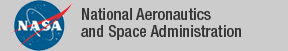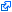Developments and Contributions

See the dependency graph of all the contributions in the NASA PVS Library NASA PVS Library 6.0.9 (11/10/14).

Development Description Contributors
ACCoRD (web) Framework for the analysis of air traffic conflict detection and resolution algorithms. AN, CM, GD, GH, JM, RB
algebra Groups, monoids, rings, etc. DL, RB
analysis Real analysis, limits, continuity, derivatives, integrals. AN, BD, RB
Bernstein (web) Formalization of multivariate Bernstein polynomials. AN, CM
complex Complex numbers.DL
complex_alt Alternative formalization of complex numbers. DL
complex_integration Complex integration.DL
co_structures Sequences of countable length defined as coalgebra datatypes.JJ
digraphs Directed graphs: circuits, maximal subtrees, paths, DAGs. AA, JS, KR, RB
exact_real_arith Exact real arithmetic including trig functions.DL
extended_nnreal Extended non-negative reals.DL
fault_tolerance Fault tolerance protocols. AG, JM, LP, PM
float Floating point numbers and arithmetic. PM, SB
graphs Graph theory: connectedness, walks, trees, Menger's Theorem. RB, JS
groups Group theory.ALG
ints Integer division, gcd, mod, prime factorization, min, max. AG, BD, PM, RB
interval_arith (web) Interval arithmetic and numerical approximations. Includes automated strategies numerical for computing numerical approximations and interval for checking satisfiability and validity of simply quantified real-valued formulas. This development includes a formalization of Allen interval temporal logic. AN, CM, DS
lebesgue Lebesgue integral with connection to Riemann Integral.DL
linear_algebra Linear algebra.HH, GP, SO
lnexp Logarithm, exponential and hyperbolic functions. DL, RB
lnexp_fnd Foundational definitions of logarithm, exponential and hyperbolic functions. AN, DL, RB
measure_integration Sigma algebras, measures, Fubini-Tonelli Lemmas.DL
metric_space Domains with a distance metric, continuity and uniform continuity.DL
numbers Elementary number theory. AG, AN, RB
orders Abstract orders, lattices, fix points. AG, BD, JJ
power Generalized Power function (without ln/exp). DL
probability Probability theory.DL
reals Summations, sup, inf, sqrt over the reals, absolute value, etc. AN, BDV, CM, PM, RB, AI
scott Scott topology.DL
series Power series, comparison test, ratio test, Taylor's theorem. AN, DL, RB
sets_aux Powersets, orders, cardinality over infinite sets. Includes functional and relational facts based on Axiom of Choice and refinement relations based on equivalence relations. BD, DL, JJ, DS
sigma_set Summations over countably infinite sets. DL
structures Bounded arrays, finite sequences, bags, and several other structures. CM, DG, KR, LP, RB
Sturm (web) Formalization of Sturm's theorem for univariate polynomials. Includes strategies sturm and mono-poly for automatically proving univariate polynomial relations over a real interval. AN, CM, AD
Tarski (web) Formalization of Tarski's theorem for univariate polynomials. Includes strategy tarski for automatically proving systems of univaraite polynomial relations on the real line. AN, CM, AD
topology Continuity, homeomorphisms, connected and compact spaces, Borel sets/functions.DL
trig Trigonometry: definitions, identities, approximations. CM, BDV, DL, GD, HG, RB, VC, AN, JVS
TRS Term rewrite systems and Robinson unification algorithm.AA, ALG, AR, MA
TU_games Cooperative TU-games.EM
vect_analysis Limits, continuity, and derivatives of vector functions. AN, CM, RB
vectors 2-D, 3-D, 4-D, and n-dimensional vectors. AN, BDV, CM, HG, PM, RB
while Semantics for the programming language "while".DL

Major Theorems in the NASA PVS Library

Theorem Theory PVS Name Author
Cauchy-Schwarz Inequality vectors@vectorscauchy_schwartz RB
Derivative of a Power Series series@power_series_derivpowerseries_deriv RB
Fundamental Theorem of Arithmetic numbers@unique_factorization Fundamental_Theorem_Arithmetic RB
Fundamental Theorem of Calculus analysis@fundamental_theorem fundamental RB
Fundamental Theorem of Interval Arithmetic interval_arith@interval_expr Eval_fundamental CM, AN
Inclusion Theorem of Interval Arithmetic interval_arith@interval_expr Eval_inclusion CM, AN
Infinitude of Primes numbers@infinite_primesprimes_infinite RB
Integral of a Power Series series@power_series_integintegral_powerseries RB
Intermediate Value Theorem analysis@continuity_intervalintermeditate_value1 BD
Law of Cosines trig_fnd@law_cosinesLaw_Cosines CM
Mean Value Theorem analysis@derivative_propsmean_value BD
Menger's Theorem graphs@mengerhard_menger JS
Order of a Subgroup algebra@finite_grouplagrange DL
Pythagorean Property - Sine and Cosine trig_fnd@trig_basicsin2_cos2 DL
Ramsey's Theorem graphs@ramseyramseys_theorem NS
Sum of a Geometric Series series@series geometric_sum RB
Taylor's Theorem analysis@taylors Taylors RB
Trig Identities: Sum and Diff of Two Angles trig_fnd@trig_basic sin_plus, ... DL
Trig Identities: Double Angle Formulas trig_fnd@rig_basic sin2a, cos2s DL
Schroeder-Berstein Theorem orders@set_antinsymmetric inj_inj_bij JJ
Denumerability of the Rational Numbers sets_aux@countable_set countable_rat JJ
Heine Theorem and Multiary Variants analysis@uniform_continuity Heine, multiary_Heine AN
Fubini-Tonelli Lemmas measure_integration@fubini_tonelli fubini_tonelli_* DL
Knuth-Bendix Critical Pair Theorem TRS@critical_pairsCP_Theorem ALG, MA
Church-Rosser Theorem TRS@results_confluenceCR_iff_Confluent ALG, MA
Newman Lemma TRS@newman_yokouchiNewman_lemma ALG, MA
Yokouchi Lemma TRS@newman_yokouchiYokouchi_lemma ALG, MA
Robinson Unification TRS@robinsonunificationcompleteness_robinson_unification_algorithm AA, MA
Confluence of Orthogonal TRSs TRS@orthogonalityOrthogonal_implies_confluent AR, MA
Sturm's Theorem Sturm@sturmsturm AN
AA Andreia Avelar Borges, University of Brasilia, Brazil Aaron Dutle, NASA, USA Alfons Geser, HTWK Leipzig, Germany Amy Isvik, Wartburg College, USA André Galdino, Federal University of Goiás, Brazil Anthony Narkawicz, NASA, USA Ana Cristina Rocha Oliveira, University of Brasilia, Brazil Bruno Dutertre, SRI, USA Ben Di Vito, NASA, USA César Muñoz, NASA, USA David Griffioen,CWI, The Netherlands David Lester, Manchester University, UK Dragan Stosic, Ireland Érik Martin-Dorel, U. Montpellier 2 & U. of Perpignan (formerly), France Heber Herencia-Zapana, NIA, USA Jerry James, Utah State University (formerly), USA Jeff Maddalon, NASA, USA Jon Sjogren, Department of Defense, USA John Siratt, University of Arkansas at Little Rock, USA Kristin Rozier, NASA, USA Gilles Dowek, INRIA, France Geroge Hagen, NASA, USA Gilberto Perez, University of La Coruña, Spain Hanne Gottliebsen, NIA (formerly), USA Lee Pike, Galois, USA Mauricio Ayala-Rincón, University of Brasilia, Brazil Natarajan Shankar, SRI, USA Paul Miner, NASA, USA Ricky Butler, NASA, USA Silvie Boldo, INRIA, France Sam Owre, SRI, USA Víctor Carreño, NASA (formerly), USA
The tagidentifies links that are outside the NASA domain